# Test: Area Of Triangles

## 20 Questions MCQ Test Mathematics (Maths) Class 9 | Test: Area Of Triangles

Description
Attempt Test: Area Of Triangles | 20 questions in 20 minutes | Mock test for Class 9 preparation | Free important questions MCQ to study Mathematics (Maths) Class 9 for Class 9 Exam | Download free PDF with solutions
QUESTION: 1

Solution:
QUESTION: 2

### The area of quadrilateral ABCD is: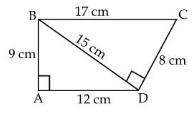​

Solution: Area of triangle abd =1/2*9*12=54 Area of triangle bcd=1/2*8*15=60 area of quadrilateral =54+60=114cm2
QUESTION: 3

### Area of an equilateral triangle is equal to

Solution:
QUESTION: 4

Diagonals AC and BD of a trapezium ABCD with AB || DC intersect each other at O. If area (ΔAOD) = 37sq cm, then area (ΔBOC) =

Solution:
QUESTION: 5

ABCD is a parallelogram whose diagonals intersect at O. A line through O intersects AB at P and DC at Q. Then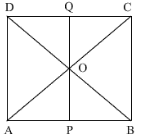Solution:
QUESTION: 6

The area of a right triangle is 30 sq cm. If the base is 5 cm, then the hypotenuse must be

Solution:
QUESTION: 7

AE is a median to side BC of triangle ABC. If area(ΔABC) = 24 cm, then area(ΔABE) =

Solution:
QUESTION: 8

D is the mid point of side BC of ABC and E is the mid point of BD. If O is the mid point of AE, then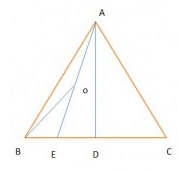Solution:
QUESTION: 9

In the figure, ∠PQR = 90°, PS = RS, QP = 12 cm and QS = 6.5 cm. The area of ΔPQR is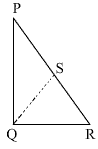Solution:
QUESTION: 10

In the given figure, if parallelogram ABCD and rectangle ABEF are of equal area then: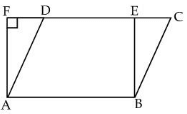Solution:
QUESTION: 11

In ΔABC, if L and M are points on AB and AC respectively such that LM BC then ar (LMC) is equal to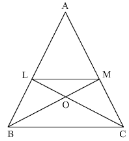Solution:
QUESTION: 12

In a parallelogram ABCD, P is a point in interior of parallelogram ABCD. If ar (||gm ABCD) = 18 cm2 then [arΔAPD) + ar(ΔCPB)] is :​

Solution:
QUESTION: 13

Diagonals AC and BD of a trapezium ABCD with AB ll DC intersect each other at O. If area (ΔAOD)=37sq cm, then area (ΔBOC) = ?

Solution:
QUESTION: 14

In ΔPQR, if D and E are points on PQ and PR respectively such that DE || QR, then ar (PQE) is equal to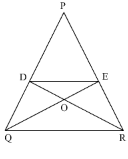Solution:
QUESTION: 15

ABC is a triangle in which D is the mid point of BC and E is the mid point of AD. Then area (ΔBED) is equal to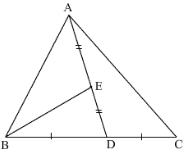Solution:
QUESTION: 16

In a parallelogram ABCD, E and F are any two points on the sides AB and BC respectively. If ar (ΔDCE) is 12 cm2, then ar(ΔADF) is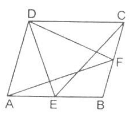Solution:
QUESTION: 17

AD is one of the medians of a Δ ABC. X is any point on AD. Then, the area of ΔABX is equal to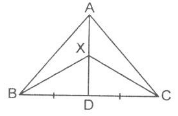Solution:
QUESTION: 18

ABC and BDE are two equilateral triangles such that D is the mid point of BC. AE intersects BC in F. Then ar (BDE) is equal to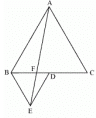Solution:
QUESTION: 19

In figure P, Q are points on sides AB, AC respectively of ΔABC such that ar (BCQ) = ar (BCP). Then,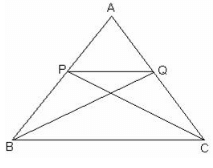Solution:
QUESTION: 20

In ΔABC, E is the mid-point of median AD. Area(ΔBED) =

Solution:

AD is the median of ΔABC. Therefore, it will divide ΔABC into two triangles of equal area.

∴ Area (ΔABD) = Area (ΔACD)

⇒Area (ΔABD ) = (1/2) area (Δ ABC) ------------(1)

In ΔABD, E is the mid-point of AD.

Therefore, BE is the median.

∴ Area (ΔBED) = Area (ΔABE)

Area (ΔBED) = (1/2)Area (ΔABD)

Area (ΔBED) = (1/2 ) x(1/2) Area (ΔABC)         [From (1)]

∴ Area (ΔBED) = (1/4)Area (ΔABC).Use Code STAYHOME200 and get INR 200 additional OFF Use Coupon Code